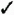# Section 80. Compute Table Totals and Subtotals

### 80. Compute Table Totals and SubtotalsBEFORE YOU BEGIN

77 Import Data into a Calc Database

78 Sort Calc Database Data

79 Filter Data That You Want to See

80. Compute Table Totals and SubtotalsWhen working with any kind of financial database information, the ability to calculate subtotals and totals, based on sorted data, becomes necessary. For example, you might want to see all the total sales from a given region or ZIP Code. If any sales are down in one area, you can get your Marketing Department to step up their efforts in that area.

Calc can summarize database data for you based on any of the following criteria:

• Sum The added total of data

• Count The number of items in the data range

• Average The calculated intermediate value in a range of data

• Min or max amounts The lowest or highest value in the data

• Product The multiplied result of the data values

• Standard deviation (of a sample or population) A statistic that measures how well dispersed values in a data range are

• Variance (of a sample or population) The square of the standard deviation used for statistical measurements

 1 Request the Summary Information Click anywhere within the data range that you want to summarize. Select Data, Subtotals to open the Subtotals dialog box. 2 Specify Summary Criteria The Subtotals dialog box opens with the 1st Group tab displayed. This is where you specify the first grouping you want to see. Often, you'll select only one group, even though Calc supports up to four subtotal groups. Specify the Group by value (such as expense category), followed by a selection of the field you want a subtotal for (such as Amount ). You then must tell Calc how the subtotals are to be calculated (such as by Sum ). In this case, you are requesting a sum for each category of expense so that you can see what you spent in each area (such as gasoline and maintenance). If you also enable the Description field and then click the Count function, Calc will tell you how many transactions are contained in each category grouping.NOTE The 2nd Group and 3rd Group tabs are for performing statistical analysis, which you may want to do when you have multiple divisions in multiple areas, or countries , and want to group first by area, then by division, and then by an individual field, such as sales per customer. The resulting summarized groups would show a subtotal of each customer, then each division, and finally each area, with a grand total at the bottom. 3 Analyze Summary Results Calc produces a summarized version of your data range. At first, the summary may look confusing because Calc inserts counts (or sums or averages or other summary items, depending on your selection) throughout your data. The count totals, for example, might show by each category in your data what the expenses were for that category and how many individual expenses there were for each category. At the end of the report, Calc provides a grand total of all the counts. 4 Collapse the Details You can click the minus sign to the left of a row number to collapse that group's detail. The minus sign then becomes a plus sign. By collapsing various types of detail in your summary (by clicking the 1, 2 , or 3 button to the left of the row number), you can get a count of the grand total only, of each group, of each group with all the details shown, respectively.Sams Teach Yourself OpenOffice.org 2, Firefox and Thunderbird for Windows All in One
ISBN: 0672328089
EAN: 2147483647
Year: 2005
Pages: 232
Authors: Greg Perry